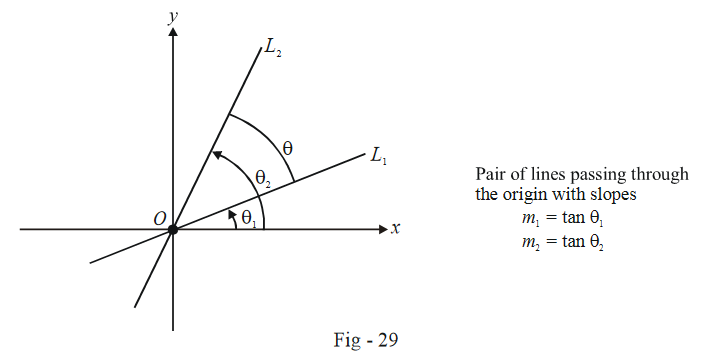# Pairs of Lines Through the origin

Go back to  'Straight Lines'

## PAIR OF LINES PASSING THROUGH THE ORIGIN

We first consider a special (and simple)case. Both the lines in our pair pass through the origin. Thus, their equations can be written as

\begin{align} {L_1}:y - {m_1}x = 0\\ {L_2}:y - {m_2}x = 0 \end{align}The joint equation of this pair is:

\begin{align} \qquad{L_1}{L_2} = 0\\ \Rightarrow \qquad & (y - {m_1}x)(y - {m_2}x) = 0\\ \Rightarrow \qquad & {y^2} + {m_1}{m_2}{x^2} - ({m_1} + {m_2})xy = 0 \qquad \qquad \qquad ...(3) \end{align}

(3) suggests that the general equation of a pair of straight lines passing through the origin is

$a{x^2} + 2hxy + b{y^2} = 0 \qquad \qquad \qquad ...(4)$

(4) is a homogenous equation of degree 2, implying that the degree of each term is 2.

It should now be apparent that any homogenous equation of degree 2 will represent two straight lines passing through the origin (we’ll soon see that the two straight lines might be imaginary; the meaning of this will become clear in a subsequent example).

Generalising, any nth degree homogenous equation of the form

${a_0}{x^n} + {a_1}{x^{n - 1}}y + {a_2}{x^{n - 2}}{y^2} + ... + {a_n}{y^n} = 0 \qquad \qquad \qquad ...(5)$

represents n straight lines (real or imaginary) passing through the origin. To obtain the slopes of these n lines, we divide by $$x^n$$ in (5) and substitute \begin{align}\frac{y}{x} = m:\end{align}

${a_n}{m^n} + {a_{n - 1}}{m^{n - 1}} + ... + {a_0} = 0$

The n values of m gives us the slopes of the n lines.